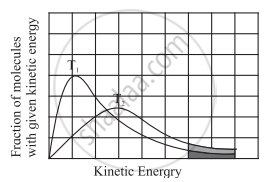Advertisement Remove all ads

# Answer the following in brief. Explain graphically the effect of temperature on the rate of reaction. - Chemistry

Answer in Brief

Answer the following in brief.

Explain graphically the effect of temperature on the rate of reaction.

Advertisement Remove all ads

#### Solution

i. The average kinetic energy of molecules is proportional to temperature.

ii. At a given temperature, the fraction of molecules with their kinetic energy equal to or greater than Ea may lead to the product.

iii. With an increase in temperature, the fraction of molecules having their energies (Ea) would increases. The rate of the reaction thus would increase.

iv. This is depicted by plotting a fraction of molecules with given kinetic energy versus kinetic energy for two different temperatures T1 and T2 (T2 > T1). The area between the curve and the horizontal axis is proportional to the total number of molecules. The total area is the same at T1 and T2.Comparison of fraction of molecules activated at T1 and T2

v. The entire shaded area which represents a fraction of molecules with kinetic energy exceeding Ea is larger at T2 than at T1 (since T2 > T1).

vi. This indicates that a fraction of molecules possessing energies larger than Ea increase with temperature. The rate of reaction increases accordingly.

Is there an error in this question or solution?
Advertisement Remove all ads

#### APPEARS IN

Balbharati Chemistry 12th Standard HSC for Maharashtra State Board
Chapter 6 Chemical Kinetics
Exercise | Q 3.15 | Page 137
Advertisement Remove all ads
Advertisement Remove all ads
Share
Notifications

View all notifications

Forgot password?